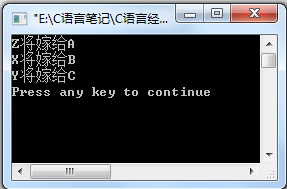# c语言实例--婚礼上的谎言

第一步：    我们设a=1 代表（a与x结婚），否则 a!=1;

b=2 代表（b与y结婚），否则b!=2;

c=3 代表（c与z结婚），否则c!=3.

第二步：现在我们知道了题目中的三种情况为假： a说他将和x结婚 代表 a!=1;

x说她的未婚夫是c 代表c!=1;

c说他将和z结婚 代表 c!=3.

第三步：根据现实情况，我们知道：3个新郎之间不能互为配偶，即代表 a!=b;a!=c;b!=c.

第四步：穷举所有的情况，再进行判断。

#include <stdio.h>                                            //引用头文件
main()
{
int a,b,c;			                      //定义新郎a,b,c三个变量
for(a=1;a<=3;a++)                                     //穷举a的所有可能
for(b=1;b<=3;b++)		              //穷举b的所有可能
for(c=1;c<=3;c++)                     //穷举c的所有肯能
if(a!=1 && c!=1 && c!=3 && a!=b && a!=c && b!=c)   //最重要的：看思路
{
printf("%c将嫁给A\n",'X'+a-1);	//输出结果：'X'+a-1 表示  字母'X'+a(最终符合条件的a)-1；
printf("%c将嫁给B\n",'X'+b-1);              'X'+b-1 表示  字母'X'+b(最终符合条件的b)-1；
printf("%c将嫁给C\n",'X'+c-1);              'X'+c-1 表示  字母'X'+c(最终符合条件的c)-1;
}
}